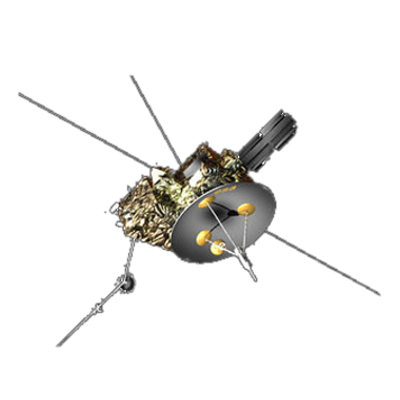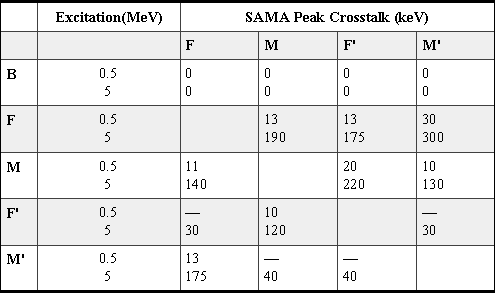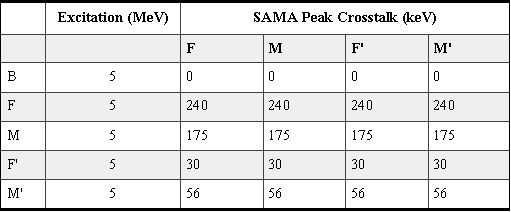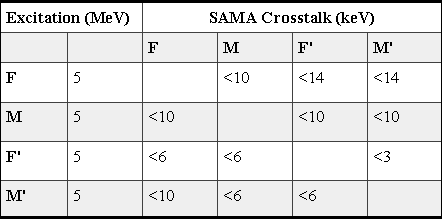ULYSSES

Ulysses HISCALE Data Analysis Handbook

## Appendix 17. LAN Analog Electronics Engineering Memos

### A17.4 LAN Spare - LEMS/LEFS LINEAR SAMA Crosstalk

Document Reference: Memo from D. E. Fort to R. E. Gold, August 28, 1984

Reference: S1I-1-036, "LAN Spare - LEMS/LEFS LINEAR Crosstalk" dated August 28, 1984 (Section A17.3)

Table A17-2 gives the test results for crosstalk in the SAMA channel. These tests were accomplished by injecting a fixed energy signal into a linear channel (with the Ca1 input shorted), and observing the output of the SAMA as each of the remaining linear channels was selected by the SAMA multiplexer. Obviously, the crosstalk is significant. Figure A17-9 shows the SAMA output waveform for two of the crosstalk cases.

Since there is no significant crosstalk in the linear channels at 5 MeV (see Section A17.3), the crosstalk must arise within the SAMA channel itself. An additional test was conducted with the SAMA multiplexer inhibited (all switches open). The results are shown in Table A17-3. Figure A17-10 shows the SAMA ouput waveform when channel F is excited. Even with all switches open, the SAMA output is significant.

Table A17-2 SAMA crosstalkTable A17-3 SAMA crosstalk, MUX inhibitedFigure A17-9 SAMA output waveform for 2 of the crosstalk casesFigure A17-10 SAMA output waveform when channel F is excitedThe crosstalk problem apparently arises due to parasitic capacitance within the multiplexer, and to the presence of a large series resistance in the signal path. For example, Figure A17-11a shows channel M' selected by the multiplexer, and Figure A17-11b shows the equivalent circuit assuming a simple shunt capacitance across each switch. F is taken to be the excited channel. A significant amount of the F signal could be coupled to the output depending on the value of the shunt capacitance.

Figure A17-11a MUX configuration with M' selectedFigure A17-11b Equivalent circuit assuming feedthrough capacitanceUnfortunately, the magnitude of the crosstalk actually observed is not in agreement with this simple model using typical values of shunt capacitance given by the manufacturer (0.2 pF). Nevertheless, the model suggests a possible solution which does in fact work. Suppose the series resistance in the example of Figure A17-11 is changed to 100W. Now the effective impedance at the multiplexer output is 100 +RS (RS = switch ON resistance, approx. 150W) instead of 10K, and the crosstalk should decrease by nearly two orders of magnitude. Table A17-4 shows the approximate values of crosstalk actually measured for this configuration. (More precise measurements will have to wait for a gain change in the log stage. See item 2 below.)

Table A17-4 Approximate crosstalk w/100WThe penalties for making such a change are as follows:

1. The large values of series resistance made independent adjustment of channel gain possible. The gains were matched so that a single SAMA response curve would serve for all channels. Making the resistance 100W eliminates this adjustment.
2. The series resistance and the shunt resistance at the multiplexer output formed a divider which reduced signal levels 45%. This will now have to be accomplished at the log stage input by increasing the value of the 1.0K input resistor to approximately 2.21K.
3. The series resistance protected the linear channels against shorts within the multiplexer.

Of these, the only serious consideration is item 1, and it should be noted that for SN003, the relative gains of the linear channels compare as follows (taking F1 as the standard):

F1 = 100%
M1 = 99+%
F1' = 99+%
M1' = 96%

Thus, the response curves for F1, M1 and F1' would be virtually identical, and the curve for M1' would be offset by only 5mV.

It should be noted that while a change to 100W drastically reduces crosstalk between channels when a channel is selected, the feedthrough with all switches open (multiplexer inhibited) will remain virtually unchanged from the values given in Table A17-3. However, since the PHA is also inhibited during these periods, no problem is expected.

Conclusion: Based on the above tests, it is recommended that the series resistance into the SAMA multiplexer (R121, R122, R123, R124) be reduced from its present value of approximately 20K to a new value of approximately 100W. In addition, the log amp input resistor must be changed from 1.0K to 2.21K to compensate for the change in the resistive divider ratio.